JEE  >  Part Test - 13 (JEE Advanced)

# Part Test - 13 (JEE Advanced)

Test Description

## 60 Questions MCQ Test JEE Main & Advanced Mock Test Series | Part Test - 13 (JEE Advanced)

Part Test - 13 (JEE Advanced) for JEE 2023 is part of JEE Main & Advanced Mock Test Series preparation. The Part Test - 13 (JEE Advanced) questions and answers have been prepared according to the JEE exam syllabus.The Part Test - 13 (JEE Advanced) MCQs are made for JEE 2023 Exam. Find important definitions, questions, notes, meanings, examples, exercises, MCQs and online tests for Part Test - 13 (JEE Advanced) below.
 1 Crore+ students have signed up on EduRev. Have you?
Part Test - 13 (JEE Advanced) - Question 1

### In the Hofmann bromamide degradation reaction, the number of moles of NaOH and Br2 used per mole of amine produced are

Part Test - 13 (JEE Advanced) - Question 2

### In the chemical reactions,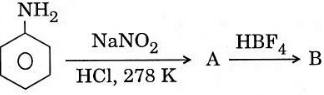Part Test - 13 (JEE Advanced) - Question 3

### A long capillary tube of mass 'π' gm,  radius 2mm and negligible thickness, is partially immersed in a liquid of surface tension 0.1 N/m. Take angle of contact zero and neglect buoyant force of liquid. The force required to hold the tube vertically, will be - (g = 10 m/s2)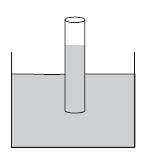Detailed Solution for Part Test - 13 (JEE Advanced) - Question 3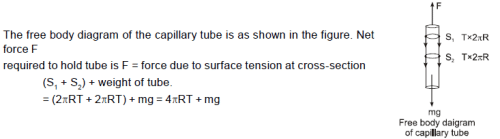Part Test - 13 (JEE Advanced) - Question 4

In the chemical reaction, CH3CH2NH2 + CHCl3 + 3KOH ⟶ (A) + (B) + 3H2O, the compounds (A) and (B) are respectively

Part Test - 13 (JEE Advanced) - Question 5

A nonuniform sphere at rest on a rough horizontal surface is acted upon by a force F as shown. The friction force acting on it is
(1) towards left        (2) towards right        (3) zero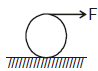Detailed Solution for Part Test - 13 (JEE Advanced) - Question 5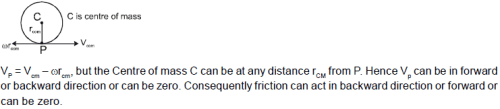Part Test - 13 (JEE Advanced) - Question 6

During synaptic transmission of nerve impulse, neurotransmitter (P) is released from synaptic vesicles by the action of ions (Q). Choose the correct P and Q.

Part Test - 13 (JEE Advanced) - Question 7

E. Coli about to replicate was placed in a medium containing radio active thymidine for five minutes. Then it was made to replicate in a normal medium. Which of the following observation shall be correct?

Part Test - 13 (JEE Advanced) - Question 8

To determine the coefficient of friction between a rough surface and a block, the surface is kept inclined at 45° and the block is released from rest. The block takes a time in moving a distance d. The rough surface is then replaced by a smooth surface and the same experiment is repeated. The block now takes a time t/2 in moving down the same distance d. The coefficient of friction is

Part Test - 13 (JEE Advanced) - Question 9

Consider a boy on a trolley who throws a ball with speed 20 m/s at an angle 37° with respect to trolley in direction of motion of trolley which moves horizontally with speed 10 m/s then what will be distance travelled by ball parallel to road :

Detailed Solution for Part Test - 13 (JEE Advanced) - Question 9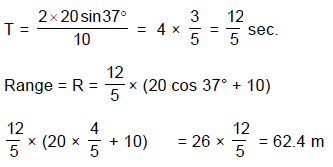Part Test - 13 (JEE Advanced) - Question 10

A block is pushed momentarily on a horizontal surface with initial velocity ‘v’. If ‘μ’ is the coefficient of sliding friction between the block and surface, the block will come to rest after time (‘g’ = acceleration due to gravity)

Part Test - 13 (JEE Advanced) - Question 11

Three blocks A, B and C of masses 4 kg, 2 kg and 1 kg respectively, are in contact on a frictionless surface, as shown. If a force of 14 N is applied on the 4 kg block, then the contact force between A and B is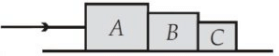*Multiple options can be correct
Part Test - 13 (JEE Advanced) - Question 12

A closed vessel contains a mixture of two diatomic gases A and B. Molar mass of A is 16 times that of B and mass of gas A, contained in the vessel is 2 times that of B. :

Detailed Solution for Part Test - 13 (JEE Advanced) - Question 12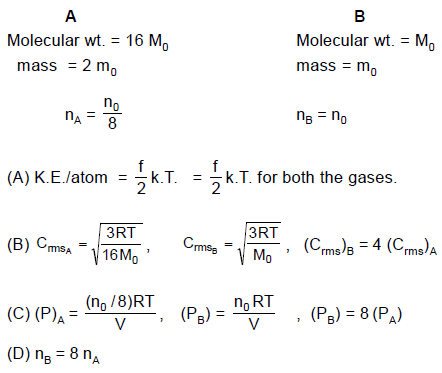Part Test - 13 (JEE Advanced) - Question 13

A plank with a box on it at one end is gradually raised about the other end. As the angle of inclination with the horizontal reaches 30º, the box starts to slip and slides 4.0 m down the plank in 4.0 s. The coefficients of static and kinetic friction between the box and the plank will be, respectively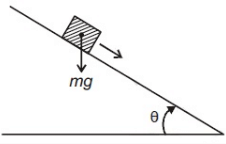*Multiple options can be correct
Part Test - 13 (JEE Advanced) - Question 14

In a resonance tube experiment, a closed organ pipe of length 120 cm resonates when tuned with a tuning fork of frequency 340 Hz. If water is poured in the pipe then (given vair = 340 m/sec.) :

Detailed Solution for Part Test - 13 (JEE Advanced) - Question 14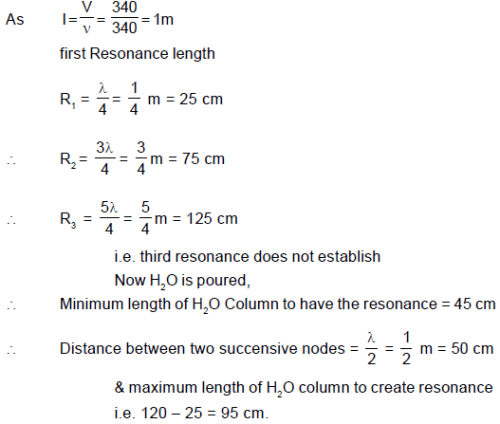Part Test - 13 (JEE Advanced) - Question 15

The force acting on a particle of mass is indicated by the force-time graph shown below. The change in momentum of the particle over the time interval from zero to 8 s is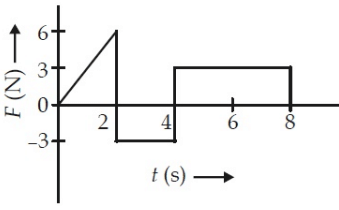Detailed Solution for Part Test - 13 (JEE Advanced) - Question 15

In standing waves, particles may have phase differences only 0 or π

*Answer can only contain numeric values
Part Test - 13 (JEE Advanced) - Question 16

A particle moving on a smooth horizontal surface strikes a stationary wall. The angle of strike is equal to the angle of rebound & is equal to 37° and the coefficient of restitution with wall is e = 1/5. Find the friction coefficient between wall and the particle in the form X/10 and fill value of X.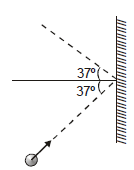Detailed Solution for Part Test - 13 (JEE Advanced) - Question 16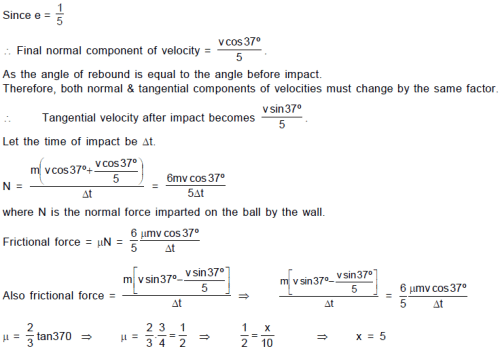*Answer can only contain numeric values
Part Test - 13 (JEE Advanced) - Question 17

A spool of mass M = 3 kg and radius R = 20 cm has an axle of radius r = 10 cm around which a string is wrapped. The moment of inertia about an axis perpendicular to the plane of the spool and passing through the centre is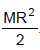Coefficient of friction between the surface and the spool is 0.4. If the maximum value of the tension is T (in N) that can be applied so that the spool rolls without slipping, then find T/2 [Take g = 10 m/s2.]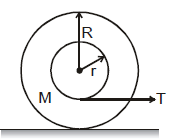Detailed Solution for Part Test - 13 (JEE Advanced) - Question 17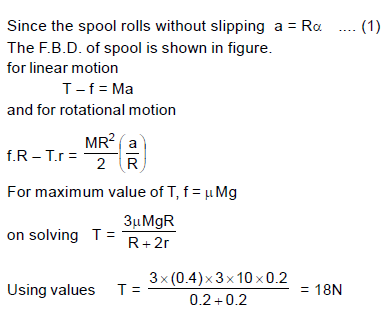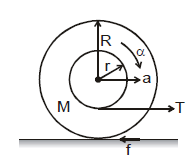*Answer can only contain numeric values
Part Test - 13 (JEE Advanced) - Question 18

A particle is projected at an angle 60º with speed 10√3 , from the point ' A ' as shown in the fig. At the same time the wedge is made to move with speed 10√3 towards right as shown in the figure. Then the time (in seconds) after which particle will strike the wedge is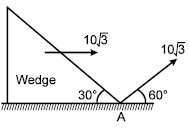Detailed Solution for Part Test - 13 (JEE Advanced) - Question 18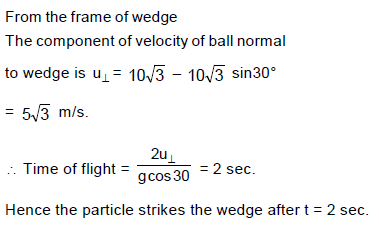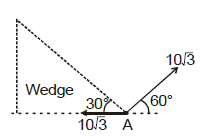*Answer can only contain numeric values
Part Test - 13 (JEE Advanced) - Question 19

In a tank of horizontal cross-sectional area 1m2, a spring with force constant 2000 Nm–1 is fixed in vertical position upto the height of the water as shown in figure 1. A block of mass 180 kg is gently placed over the spring and it attains the equilibrium position as shown in figure 2. If base area of the block is 0.2m2 and height 60 cm, then compression in the spring is 5X (in cm)  in equilibrium position. Then find X (take g = 10 m/s2; ρw = 1000 kg/m3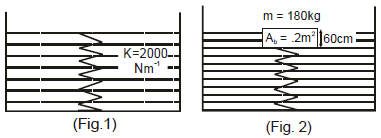Detailed Solution for Part Test - 13 (JEE Advanced) - Question 19

As block goes down by distance x, water comes up by distance y. As both are measured from initial level of water, compression in the spring is x but the block is in depth (x + y) in water. Applying conservation of volume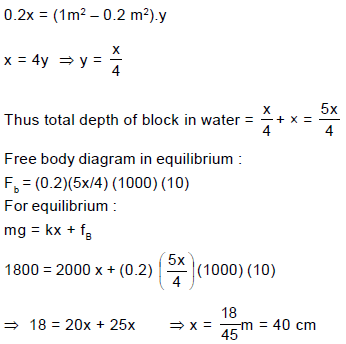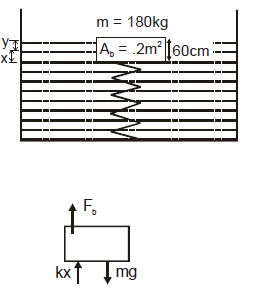*Answer can only contain numeric values
Part Test - 13 (JEE Advanced) - Question 20

A planet revolves about the sun in elliptical orbit of semimajor axis 2 × 1012 m. The areal velocity of the planet when it is nearest to the sun is 4.4 × 1016 m2/s. The least distance between planet and the sun is 1.8 × 1012 m. The minimum speed of the planet is a × 101 (in km/sec) then find a

Detailed Solution for Part Test - 13 (JEE Advanced) - Question 20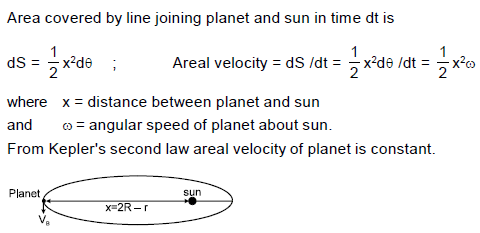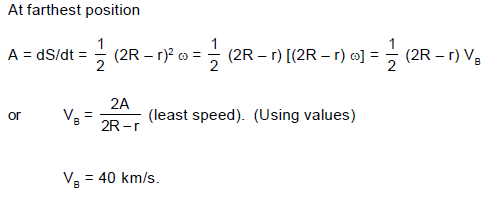Part Test - 13 (JEE Advanced) - Question 21

A light spring balance hangs from the hook of the other light spring balance and a block of mass kg hangs from the former one. Then the true statement about the scale reading is

Part Test - 13 (JEE Advanced) - Question 22

A roller is made by joining together two cones at their vertices O. It is kept on two rails AB and CD which are placed asymmetrically (see figure), with its axis perpendicular to CD and its centre O at the centre of line joining AB and CD (see figure). It is given a light push so that it starts rolling with its centre O moving parallel to CD in the direction shown. As it moves, the roller will tend to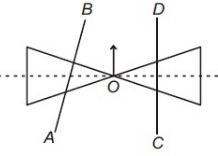Part Test - 13 (JEE Advanced) - Question 23

A block of mass m is placed on a smooth wedge of inclination θ. The whole system is accelerated horizontally, so that the block does not slip on the wedge. The force exerted by the wedge on the block (g is acceleration due to gravity) will be :

Part Test - 13 (JEE Advanced) - Question 24

A horizontal force of 10 N is necessary to just hold a block stationary against a wall. The coefficient of friction between the block and the wall is 0.2. The weight of the block is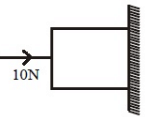Detailed Solution for Part Test - 13 (JEE Advanced) - Question 24

For H+ cation is smaller than parent H-atom. H and Li+ are isoelectronic species so Li+ with higher nuclear charge (i.e. 3) will be smaller than H having smaller nuclear charge (i.e.1).

Part Test - 13 (JEE Advanced) - Question 25

BF3 & CCl4 on reaction with water produce _________ & _________ respectively :

Detailed Solution for Part Test - 13 (JEE Advanced) - Question 25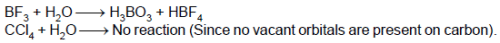Part Test - 13 (JEE Advanced) - Question 26

In manufacture of sodium carbonate from solvay (or ammonia soda) process the raw material used is :

Detailed Solution for Part Test - 13 (JEE Advanced) - Question 26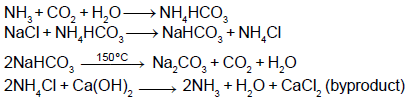Part Test - 13 (JEE Advanced) - Question 27

For the following flow diagram :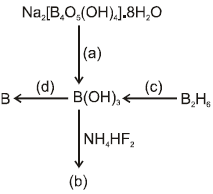Which of the following option describes the reagents, products and the reaction conditions given in parentheses as small alphabets ?

Detailed Solution for Part Test - 13 (JEE Advanced) - Question 27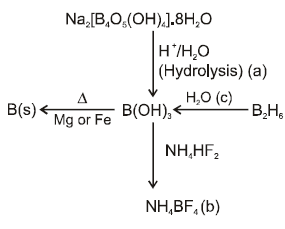Part Test - 13 (JEE Advanced) - Question 28

The acceptable resonating structures of the following molecule are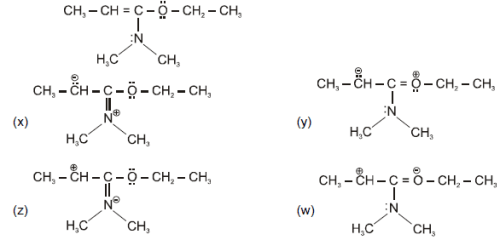Detailed Solution for Part Test - 13 (JEE Advanced) - Question 28

x & y are proper resonating structures while z & w violate octet rule.

Part Test - 13 (JEE Advanced) - Question 29

Which is not the correct order as per property given for the following pair of compounds:

Detailed Solution for Part Test - 13 (JEE Advanced) - Question 29

Negative charge is stabilised on more electronegative atom.

Part Test - 13 (JEE Advanced) - Question 30

Chiral molecule should not have ?

Detailed Solution for Part Test - 13 (JEE Advanced) - Question 30

Definition based.

*Multiple options can be correct
Part Test - 13 (JEE Advanced) - Question 31

Choose the correct statement :

Detailed Solution for Part Test - 13 (JEE Advanced) - Question 31

Use equivalent of oxidising agent = equivalents of reducing agent.

*Multiple options can be correct
Part Test - 13 (JEE Advanced) - Question 32

The solubility of a sparingly soluble salt Ax By in water at 25ºC = 1.4 × 10–4 M. The solubility product is 1.1 × 10–11. The possibilities are

Detailed Solution for Part Test - 13 (JEE Advanced) - Question 32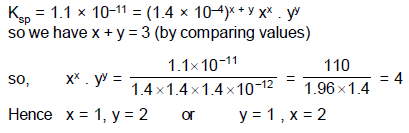*Multiple options can be correct
Part Test - 13 (JEE Advanced) - Question 33

Which of the following property(ies) of alkali metals/alkali metal salts increase(s) with increase in atomic number?

Detailed Solution for Part Test - 13 (JEE Advanced) - Question 33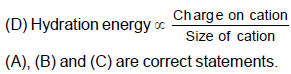Part Test - 13 (JEE Advanced) - Question 34

Suppose the elements X and Y combine to form two compounds XY2 and X3Y2. When 0.1 mole of XY2 weighs 10 g and 0.05 mole of X3Y2 weighs 9 g, the atomic weights of X and Y are

Part Test - 13 (JEE Advanced) - Question 35

The energies of activation for forward and reverse reactions for A2 + B2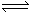2AB are 180 kJ mol–1 and 200 kJ mol–1 respectively. The presence of a catalyst lowers the activation energy of both (forward and reverse) reactions by 100 kJ mol–1. The enthalpy change of the reaction (A2 + B2 → 2AB) in the presence of catalyst will be (in kJ mol–1):

*Answer can only contain numeric values
Part Test - 13 (JEE Advanced) - Question 36

The number of visible lines when an electron returns from the 5th orbit to ground state in the hydrogen spectrum is P. Then value of P is :

Detailed Solution for Part Test - 13 (JEE Advanced) - Question 36

No. of visible lines means Balmer series
Hence, n – 2 = 5 – 2 = 3.

*Answer can only contain numeric values
Part Test - 13 (JEE Advanced) - Question 37

A spherical balloon of volume 5 litre is to be filled up with H2 at NTP from a cylinder of 6 litre volume containing the gas at 6 atm at 0°C. The no. of balloons that can be filled up is :

Detailed Solution for Part Test - 13 (JEE Advanced) - Question 37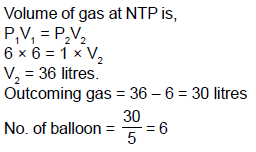*Answer can only contain numeric values
Part Test - 13 (JEE Advanced) - Question 38

For the equilibrium reaction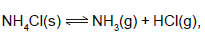Kp=81 atm2 The total pressure at equilibrium is 'X' times the pressure of NH3. The value of X will be :

Detailed Solution for Part Test - 13 (JEE Advanced) - Question 38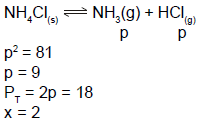*Answer can only contain numeric values
Part Test - 13 (JEE Advanced) - Question 39

How many structure isomers of primary amines are possible with molecular formula C5H13N ?

Detailed Solution for Part Test - 13 (JEE Advanced) - Question 39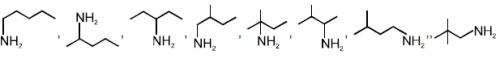*Answer can only contain numeric values
Part Test - 13 (JEE Advanced) - Question 40

What is the minimum pH required to prevent the precipitation of ZnS in a solution that is 0.01M ZnCl2 and saturated with 0.10 M H2S ? Given Ksp of ZnS = 10–21, for H2S Ka1 x Ka2 = 10-20 .

Detailed Solution for Part Test - 13 (JEE Advanced) - Question 40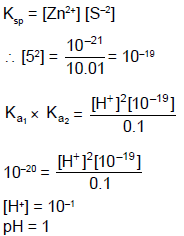Part Test - 13 (JEE Advanced) - Question 41

If x1, x2, x3, x4, x5, .... is a Geometric Progression of natural number and x1x2x3x4 = 64, then complete set of solutions of x4 is

Detailed Solution for Part Test - 13 (JEE Advanced) - Question 41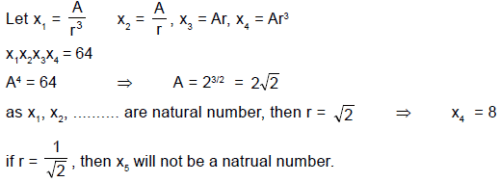Part Test - 13 (JEE Advanced) - Question 42

From a point P = (3, 4) perpendiculars PQ and PR are drawn to line 3x + 4y – 7 = 0 and  a variable line y – 1 = m (x – 7), then maximum area ΔPQR is

Detailed Solution for Part Test - 13 (JEE Advanced) - Question 42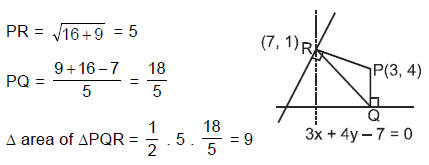Part Test - 13 (JEE Advanced) - Question 43

If  P(x1, y1), Q(x2, y2) be any two points on the curve y = 3x – 2 – x2 for 1 < x < 2, then maximum value of 3x1 + 3x2 – x12 – x22  is

Detailed Solution for Part Test - 13 (JEE Advanced) - Question 43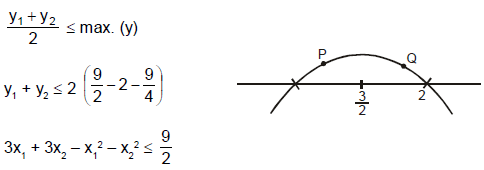Part Test - 13 (JEE Advanced) - Question 44

A point O is situated on a circle of radius R and with centre O another circle of radius  3R/2 is described. Inside the smaller crescent-shaped region intercepted between these circles a circle of radius R/8 is placed. If smaller circle moves in contact with the circle of radius R, then the length of the arc described by its centre in moving from one extreme position to other extreme position is

Detailed Solution for Part Test - 13 (JEE Advanced) - Question 44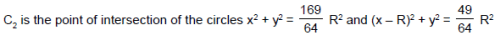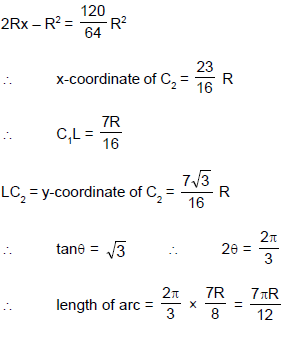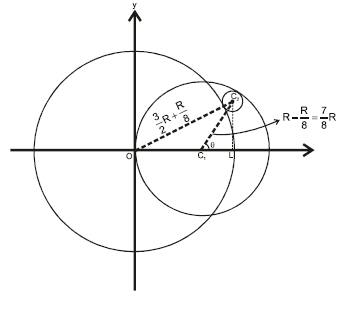Part Test - 13 (JEE Advanced) - Question 45

If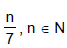is a non terminating and is a recurring decimal rational number. After decimal at some stage, digits start repeating in groups continuously. If smallest such group has r digits, then

Detailed Solution for Part Test - 13 (JEE Advanced) - Question 45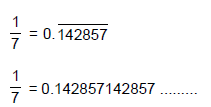Clearly there are 6 digits in repeating group.
A repeating group can not contain 7 or more than 7 digits because the divisor is 7.
Hence maximum value of r is 6.

Part Test - 13 (JEE Advanced) - Question 46

All the three vertices of an equilateral triangle lie on the parabola  y = x2  and one of its sides has a slope of 2, then sum of x-coordinates of the three vertices of triangle is

Detailed Solution for Part Test - 13 (JEE Advanced) - Question 46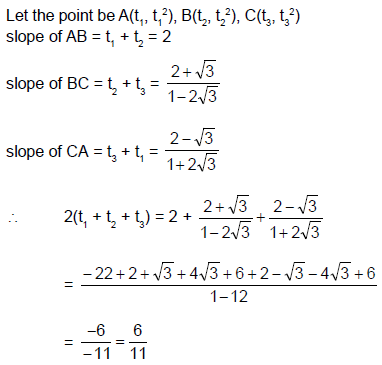Part Test - 13 (JEE Advanced) - Question 47

The number of common tangents to the hyperbola xy = c2  and the circle  x2 + y2 = 2c2  is

Detailed Solution for Part Test - 13 (JEE Advanced) - Question 47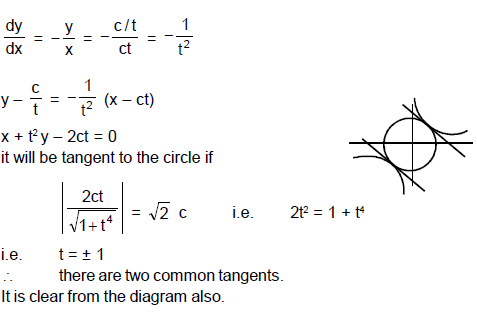Part Test - 13 (JEE Advanced) - Question 48

If x1 x2 x3 = 2.5.72, then the number of different solutions for the ordered triplets (x1, x2, x3) where xi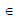N, xi > 1 is

Detailed Solution for Part Test - 13 (JEE Advanced) - Question 48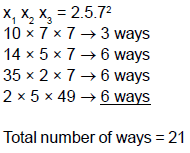Part Test - 13 (JEE Advanced) - Question 49

Consider the reaction,

2A + B → Products

When concentration of B alone was doubled, the half-life did not change. When the concentration of A alone was doubled, the rate increased by two times. The unit of rate constant for this reaction is:

Part Test - 13 (JEE Advanced) - Question 50

If f(x) = cosec 2x + cosec 22x + .......... + cosec 2nx and g(x) = f(x) + cot 2nx, then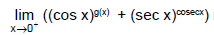is equal to

Detailed Solution for Part Test - 13 (JEE Advanced) - Question 50

g(x) = cosec 2x + cosec22x + ....... + cosec2nx + cot 2nx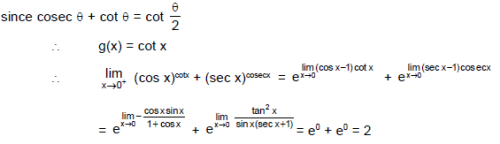Part Test - 13 (JEE Advanced) - Question 51

Consider a reaction, aG + bH → Products.

When concentration of both the reactants G and H is doubled, the rate increases by eight times. However, when the concentration of G is doubled keeping the concentration of H fixed, the rate is doubled. The overall order of the reaction is:

Part Test - 13 (JEE Advanced) - Question 52

The mass of carbon anode consumed (giving only carbon dioxide) in the production of 270 kg of aluminium metal from bauxite by the Hall process is:

(Atomic mass : Al = 27)

*Multiple options can be correct
Part Test - 13 (JEE Advanced) - Question 53

If z1 = a + ib & z2 = c + id are complex numbers such that |z1| = |z2| = 1 and
Re (z1 z2) = 0, then the pair of complex numbers w1 = a + ic & w2 = b + id satisfies :

Detailed Solution for Part Test - 13 (JEE Advanced) - Question 53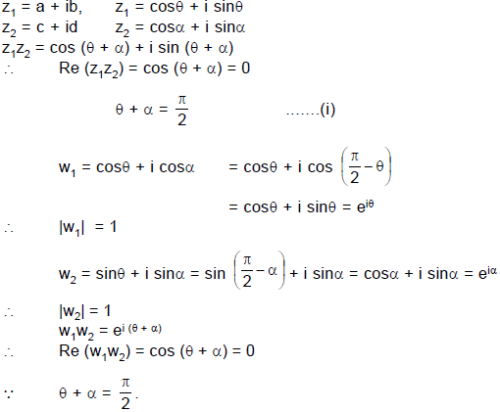*Multiple options can be correct
Part Test - 13 (JEE Advanced) - Question 54

If z is a complex number satisfying |z – i Re (z) | = |z – Im (z)| then z lies on

Detailed Solution for Part Test - 13 (JEE Advanced) - Question 54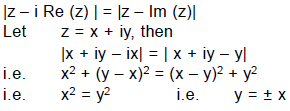Part Test - 13 (JEE Advanced) - Question 55

Concentrated aqueous sulphuric acid is 98% H2SO4 by mass and has a density of 1.80 g.mL–1. Volume of acid required to make 1 litre of 0.1 M H2SO4 solution is:

Detailed Solution for Part Test - 13 (JEE Advanced) - Question 55
We need 9.8 gram H2SO4Convert this mass in volume using density = mass/volume1.80 gml-1 = 9.8g /volumevolume = 9.8 g/1.8g ml-1= 5.44 ml.5.44 ml will require if solution is 100 % pure, But given it is 98%So the volume required = 100 x 5.44/98= 5.55 ml
The volume of acid required to make 1 litre of 0.1 M H2SO4
*Answer can only contain numeric values
Part Test - 13 (JEE Advanced) - Question 56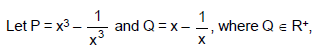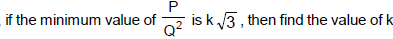Detailed Solution for Part Test - 13 (JEE Advanced) - Question 56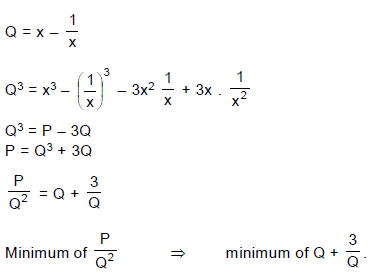Here product of numbers Q and 3/Q
is constant so sum of these numbers will be minimum if these are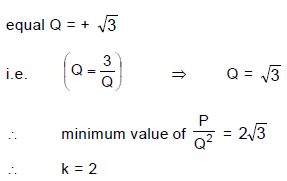*Answer can only contain numeric values
Part Test - 13 (JEE Advanced) - Question 57

If in a triangle ABC,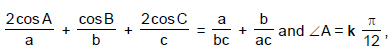then find the value of k.

Detailed Solution for Part Test - 13 (JEE Advanced) - Question 57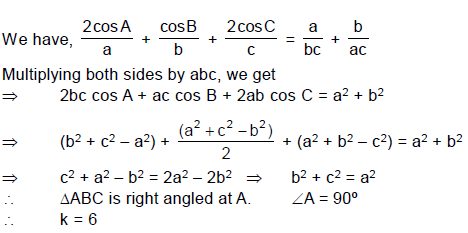*Answer can only contain numeric values
Part Test - 13 (JEE Advanced) - Question 58

The tangent at any point P of  a curve C meets the x - axis at Q whose abscissa is positive and OP = OQ O being the origin, if C is a family of parabolas having vertix (α, β) and latus rectum = 4a, then evaluate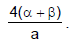Detailed Solution for Part Test - 13 (JEE Advanced) - Question 58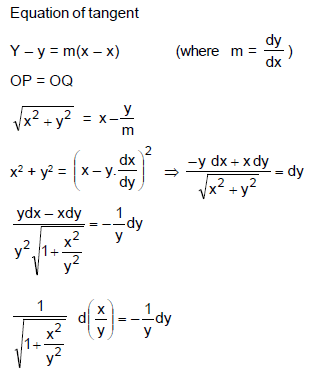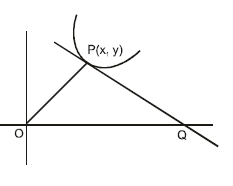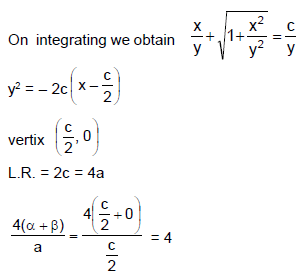*Answer can only contain numeric values
Part Test - 13 (JEE Advanced) - Question 59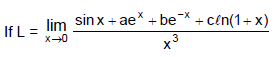exists then find the value of (a + b + c).

Detailed Solution for Part Test - 13 (JEE Advanced) - Question 59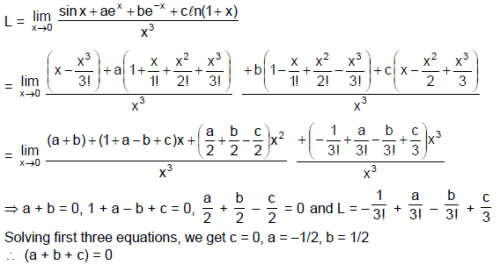*Answer can only contain numeric values
Part Test - 13 (JEE Advanced) - Question 60

Find the smallest positive integer n for which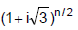is real.

Detailed Solution for Part Test - 13 (JEE Advanced) - Question 60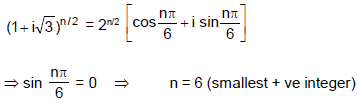## JEE Main & Advanced Mock Test Series

2 videos|327 docs|203 tests
 Use Code STAYHOME200 and get INR 200 additional OFF Use Coupon Code